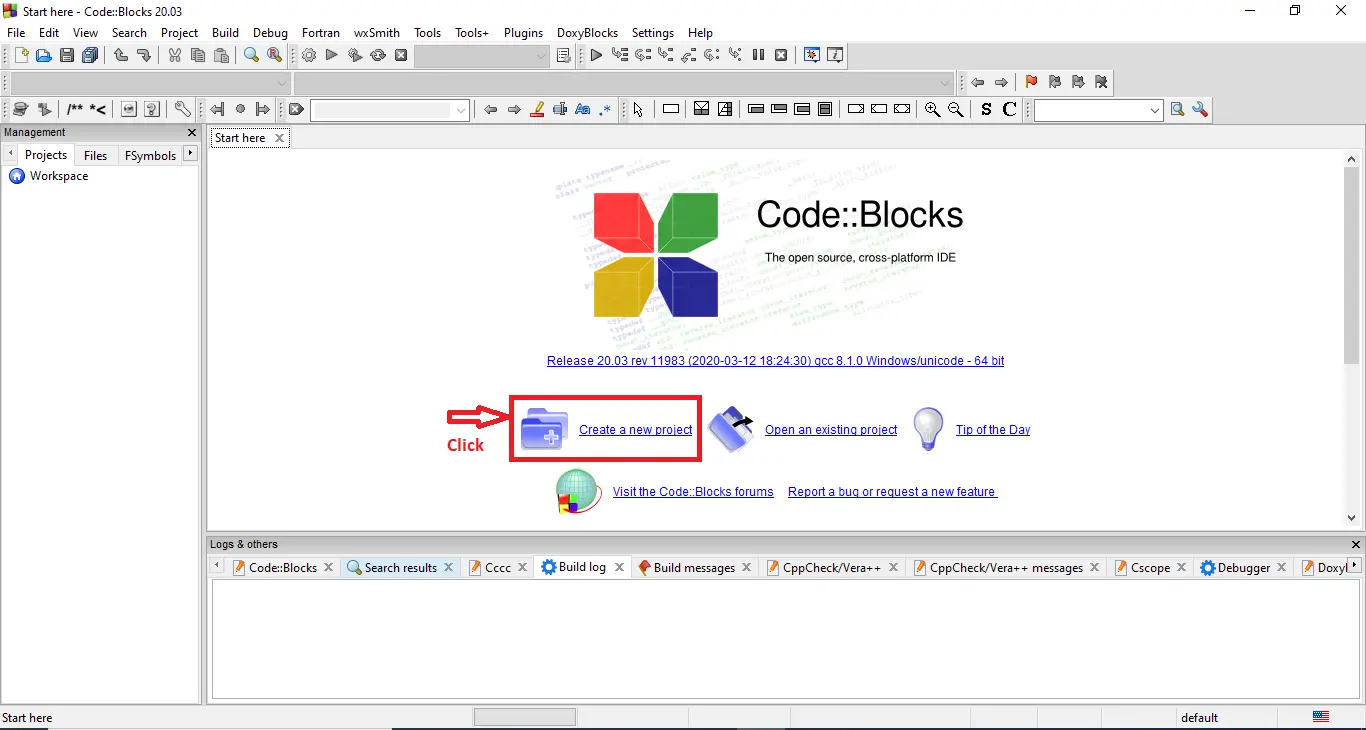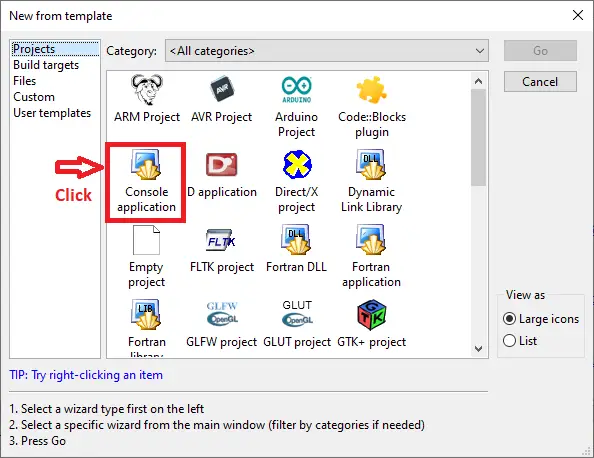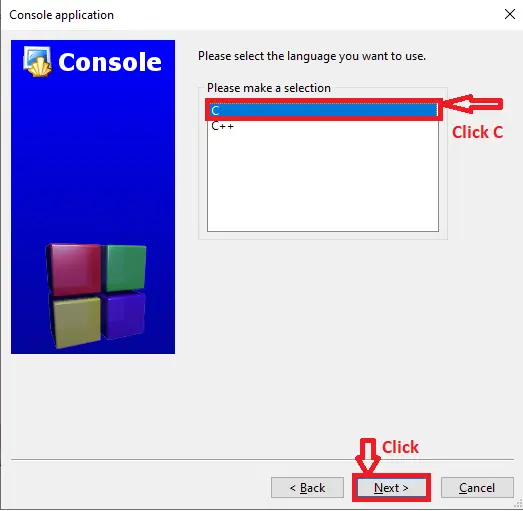# Sudoku Game Code in C Language with Source Code

## Sudoku Game Code in C Language with Source Code

This Sudoku Programming in C teaches you how to create and solve Sudoku problems using computer programs. One of the most well-known classic games is Sudoku. Sudoku uses a 99 square grid as its playing grid. The game starts with 9 squares that combine rows and columns. Every one of these nine squares is a different grid of 33 square areas.

There are 9 squares in each row and column. It can be a one-player or two-player game in which the participants aim to fill the boxes as quickly as possible. The goal of the game is to fill boxes with numbers ranging from 1 to 9 in each square. The thrilling aspect of the Sudoku game is that no number can be repeated in any column, row, or square.

Sudoku Game Code in C Language it include a free Download Source Code. Simply locate the downloadable source code below and click the “Download Now” button. To start creating a Calculator in C Language, make sure that you have a Code Blocks or any platform of C installed in your computer.

## Sudoku Game Code in C Language Steps on How to Create a Project

Time needed: 5 minutes.

Here’s the step’s on how to create a Sudoku Game Code in C Language with Source Code.

Sudoku Game in C Language with Sour...
Sudoku Game in C Language with Source Code | C Language Projects with Source Code
• Step 1: Create a new project.

First open the code blocks IDE and click “create a new project“.• Step 2: Choose console application.

Next, click the “console application” and after that click “next“.• Step 3: Choose C language.

Then , choose “C language” and click “next“.• Step 4: Name your project.

Lastly, name the project you’ve created and click “next” after that click “finish“.• Step 5: The actual code.

Finally, we will now start adding functionality to our C Framework by adding some functional codes.

## This Sudoku Game Code in C Language was build and run under Code::Blocks IDE.

• This is the code to fill the board.
```for (row = 0; row < 36; row++)
for (col = 0; col < 36; col++)
data[row][col] = 0;
```
• This is the function to check if default board is broken.
```if (copy[row][col] != 0)
{
if (checkinvalid(copy, row, col) == 1)
return -1;
}```
• Function to fill in the empty space.
```for (space = 1; space <= boardrow; space++)
{```
• Function to check if it is duplicates.
`invalidflag = checkinvalid(copy, row, col);`
• The Function for determining whether a value should go into a position(Sudoku Programming in C)
```int i, j, k;
int nonetrow, nonetcol;

for (i = 0; i < boardrow; i++)```
• Function to check to check row and columns for invalid
```if ((i == row));
else if (copy[row][col] == copy[i][col])
return 1;

if ((i == col));
else if (copy[row][col] == copy[row][i])
return 1;```
• Function to actually print data into square(Sudoku Programming in C)
```if (board[row-1][col-1] != 0)
{
if ((board[row-1][col-1]) > 9)
printf(" %d", board[row-1][col-1]);
else
printf(" %d ", board[row-1][col-1]);
}```
• The Function if it has passed or go to next level
```if (invalidflag == 0)
{
status = solver(copy, 0);

if ( status > 0)
{
return status;
}

else if ( status == -1 )
return -1;
}```
• Function to start at two after the end of “rows”(Sudoku Programming in C)
```for (i = 5; i < strlen(command); i++)
boardrow = 10*boardrow + (command[i] - '0');

switch(boardrow)
{
case 4: nonetsize = 2; break;
case 9: nonetsize = 3; break;
case 16: nonetsize = 4; break;
case 25: nonetsize = 5; break;
case 36: nonetsize = 6; break;
default:
printf("Rows/columns number must be the square of an integer.\n");
boardrow = temp;
}```